># 从 0 开始机器学习 - 线性回归 & 代价函数 & 梯度下降

## 一、机器学习问题解决流程## 二、第一个机器学习问题：单变量线性回归

### 2.1 问题描述### 2.2 训练数据集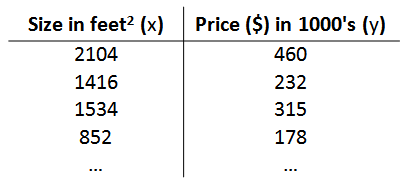• m 代表训练集中实例的数量
• x 代表输入变量（特征 Feature）
• y 代表输出变量（目标变量）
• (x, y) 代表训练集中的实例
• (Xi, Yi) 代表第 i 个观察实例
• h 代表学习算法的解决方案或函数，也称为假设函数（hypothesis

### 2.3 模型选择及总结

• 训练集：人为标记的房屋面积和对应房价的一组数据点
• 学习算法：梯度下降法
• 输入和输出：房屋的预测价格 $y = h$(房屋面积 x)
• 假设函数 $h$： $h_\theta \left( x \right)=\theta_{0}+\theta_{1}x$，线性函数拟合

## 三、代价函数（Cost Function）

### 3.1 预测房价的代价函数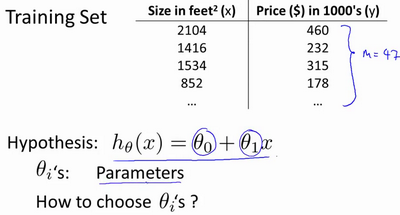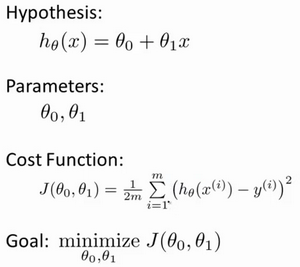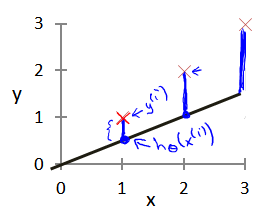### 3.2 理解 2 个参数的代价函数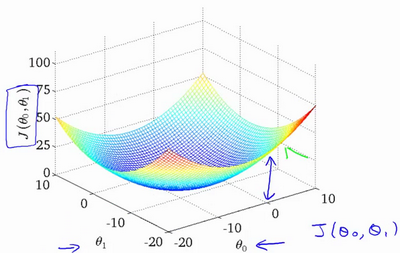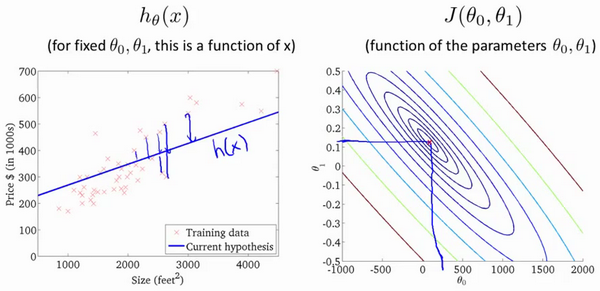• 渔网图像中（$\theta_0$，$\theta_1$）在渔网的最底部取得，使得代价函数达到最小值；
• $h_\theta \left( x \right)$ 图像中预测的函数曲线对训练数据的拟合效果达到最优；

### 4.1 算法思想### 4.2 算法数学理解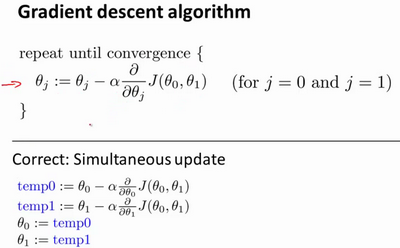• $\theta_j$ 是待更新的参数 （$\theta_0$，$\theta_1$）
• $\alpha$ 是学习率（learning rate），决定了代价函数在下降方向每次更新的步长（其实就是每次下山的小碎步）
• $\alpha \frac{\partial }{\partial {\theta_j}}J(\theta_0,\theta_1)$ 是代价函数对（$\theta_0$，$\theta_1$）的偏导数
• 因为是求偏导，所以（$\theta_0$，$\theta_1$）必须同步更新

### 4.3 算法的直观理解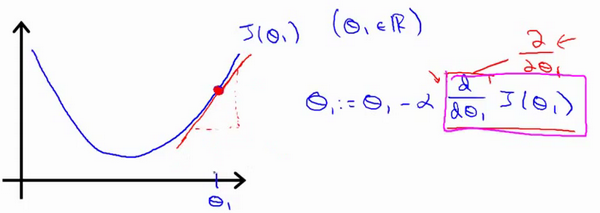• 如果 $\alpha$ 太小，每次更新的步长很小，导致要很多步才能才能到达最优点；
• 如果 $\alpha$ 太大，每次更新步长很大，在快接近最优点时，容易应为步长过大错过最优点，最终导致算法无法收敛，甚至发散；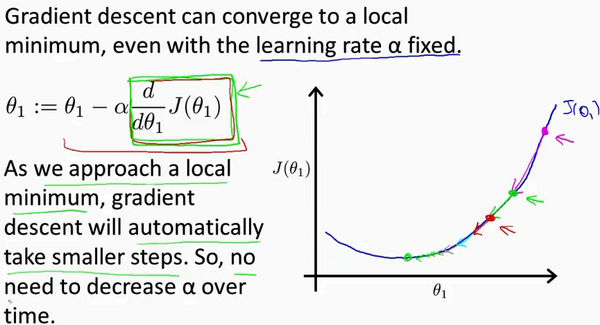## 五、梯度下降的线性回归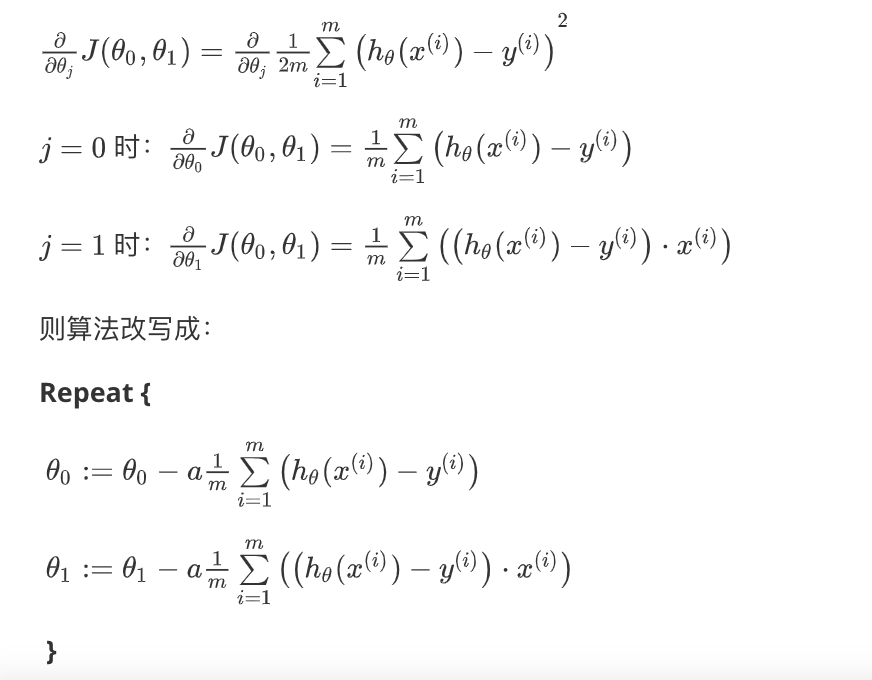DLonng at 03/21/20